# How Computer Works

## How Does the Computer Work?

Let us first discuss some of the fundamentals before learning a programming language to get awareness about a few things. Mostly in academics, these are the basic concepts that are taught. So, let us first understand what a computer is, how a computer works, and what number systems are.

##### What is a Computer?

A computer is a machine, or you can say it is an electronic device that performs operations or calculations based on instructions provided by the user (through software or hardware programs). It will accept data (input from the user), process it as per the instructions provided by the user, and then produce outputs. Computers can also store data for later use in storage devices and retrieve it whenever necessary.

##### How Does the Computer Work?

Let us discuss how the computer works. To understand how a computer works, please look at the following basic block diagram of a computer and let us try to understand the different components of a computer.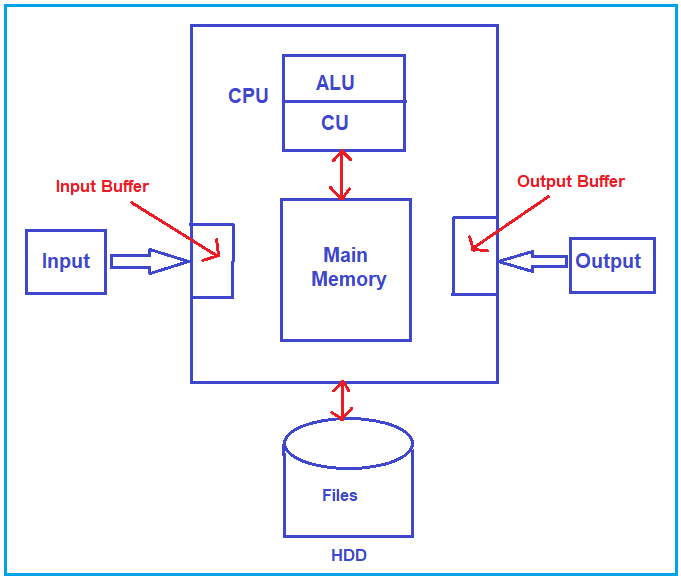Let’s study the components of a computer. The computer consists of the following components.

• CPU- Central Processing Unit.
• Main Memory
• Hard disk
• Input devices
• Output devices

Let us understand the components in detail.

##### CPU

CPU is the heart of the computer. CPU stands for Central Processing Unit or Microprocessor. The computer’s brain is the CPU, where actual data manipulation or Operations happen using ALU (Arithmetic and logical unit). Suppose you see the present-day processors like i3, i5, i7, etc. The CPU consists of two components, i.e., ALU (Arithmetic Logic Unit) and CU (Control Unit).

• ALU- Arithmetic Logic Unit: ALU performs the basic logical and arithmetic operations. Arithmetic operations like addition, subtraction, multiplication, division, and modulus. Logical operations like comparing two numbers (such as >, <, >=, <=, etc.), logical AND, OR, NOT, etc. So, ALU has performed these basic operations.
• CU- Control Unit: The control unit controls the other components like Input and output devices, main memory, hard disk, etc. The job of the control unit is to utilize other resources effectively.
##### Hard Disk:

A hard disk is a storage device for a computer. It contains two types of files, i.e., data files and program files. We can store data or programs on the hard disk; when we store something on the hard disk, it is permanent storage.

If you take the example of the notepad on our computer, it is a program file. So, when we open Notepad it’s a program, and a file is available, i.e., Program file (notepad.exe). So, basically, we click on the notepad icon, and the program will start, but if you look into My Computer and folders, somewhere you will find the program file for the notepad (C:\Windows\System32), and the file name will be notepad.exe. Now, if you type something in Notepad and save it, it will save as a text file such as MyDoc.txt, and it is a data file used by Notepad or generated by Notepad. For a better understanding, please have a look at the below diagram.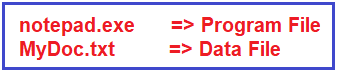Program files: The music player on the computer, notepad on the computer, and MS Office on the computer are program files. That means any application or software installed on the computer is a program file.

Data files: Movies, Songs, Word Documents, Text Documents, etc. are data files. The data files contain the actual data. If you want to perform read and write operations on the data (i.e., the data file), we need a program file.

Note: Without a program file, we cannot perform the read and write operations on the data file. For example, you have a .doc file, but if you have uninstalled MS Office from your machine, then even though you have the doc file, you cannot perform any operation on that doc file.

##### Main Memory:

Whenever the user wants to run any program (Program Files), it should be brought into the main memory so the CPU can execute it. Your Program File is stored on the HARD DISK, and the CPU cannot access the HARD Disk directly. CPU can access the Main memory.

So, the Program (Program File) needs to be brought from the Hard Disk into the Main Memory so that the CPU can execute it. This is why Main memory is also called Working Memory or Primary Memory.

Once the program is brought to the Main memory, only the CPU executes the program line by line. So, whatever program we run they are brought into the Main memory. If the program needs data from the keyboard or has to display some data on the monitor, then it can utilize the INPUT and OUTPUT devices.

##### Input Devices:

Input devices are Keyboard, Mouse, etc. These devices are used to give inputs to the computer. Input devices have their own memory in the computer called Input Buffer. All instructions from input devices are dumped into this memory, and if the program wants to read something, it will read from the input buffer. So, whatever we type using the keyboard will be stored in the input buffer, and the program reads the input from the input buffer.

##### Output Devices:

Output devices are monitors, printers, etc. Output devices show the output after the execution of the program file. Output devices also have their own memory called output buffers. If the program wants to print something, then whatever it wants to print will be put in the output buffer, which will appear on the output screen.

These are the components of a computer system, and this is how a program works inside it. You might have one question about how a computer understands the programming language or instructions. So, let’s look at it. The computer only understands the binary number system.

##### Introduction to Number System

Firstly, let us know why we need to understand the Number System while learning a programming language. The Number System we Human Beings follow is the Decimal number system, but the computer understands only the Binary Number System. So again, there is a difference between how we saw the number and how the computer saw it.

###### Example:

Firstly, let us know why we need to understand the Number System while learning a programming language. The Number System we Human Beings follow is the Decimal number system, but the computer understands only the Binary Number System. So again, there is a difference between how we saw the number and how the computer saw it.

Example: As a human, if I say 10 (one zero), for me, it is Ten, but for the computer, it is two. So, it’s necessary to understand the different types of number systems. The different types of number systems are as follows:

1. Binary Number System: {0,1}
2. Octal Number System: {0,1,2,3,4,5,6,7}
3. Decimal Number System: {0,1,2,3,4,5,6,7,8,9}
4. Hexadecimal Number System: {0,1,2,3,4,5,6,7,8,9, A, B, C, D, E, F}

Basically, we use the decimal number system, and in the decimal number system, the pictures or figures or symbols are from 0 to 9, i.e., a total of 10 symbols. As we already discussed, the computer works on zeros and ones. So, for computers, there are only two symbols, and the binary number systems have only two symbols, i.e., 0 and 1.

In the decimal number system, once we have finished with the last symbol, i.e., 9, after 9, we use a combination of symbols (from 0 to 9). For example- 10, 11, 12, and so on. 10 combines two symbols, i.e., 1 and 0. Similarly, 11 is the combination of 1 and 1.

So, the point that you need to understand is that once we finish with the symbols, we can take the combination of symbols, and in the binary number system, the same methodology is also followed. To denote the new numbers, we need to combine two symbols, i.e., 1’s and 0’s.

For example, to denote 2 in the binary system, we will combine 1 and 0, i.e., 10. Don’t read it as ten; read it as one zero. For more details, please look at the following table, which shows the numbers from 0 to 16 in the Decimal, Binary, Hexadecimal, and Octal number system.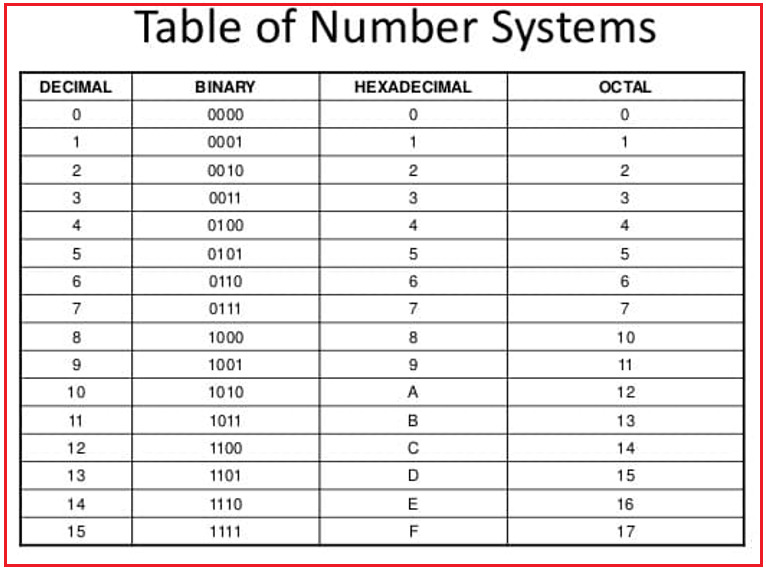The above table gives how we see numbers in each number system. However, one thing here is common: when we exceed the number, then the combination of numbers will come. For Example, in the decimal system 0 to 9 after nine, ten combines first and second, i.e., 0, and 1, i.e., 10.

##### How do we Convert Decimal Numbers to Binary Numbers?

For example- 29 is the decimal number; let’s see how we can convert this decimal number into a binary number. The base for the binary number is 2, and hence, we need to perform successive division 2, take the remainder, and then read the remainder from bottom to top to get the binary number as shown in the image below.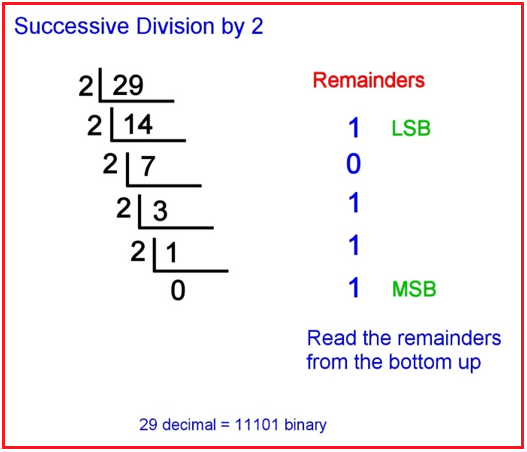This is how a decimal number is converted into binary. Now, we will see how to convert a binary number to decimal form. For example – 10101 is the binary number, and we need to find its equivalent decimal number. To better understand how to convert, please look at the following diagram.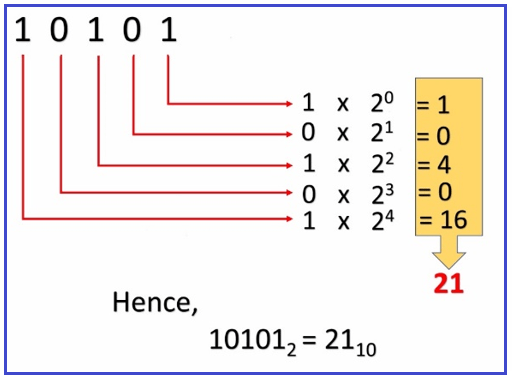So, this is how we can convert a binary number to a decimal number. We have seen how to convert decimal numbers to binary and binary numbers back to decimals.

In the next article, I will briefly introduce Programming Languages. Here, in this article, I try to explain how a computer works in detail, and I hope you enjoy this How does the computer work article.

### 2 thoughts on “How Computer Works”

1.Interesting. I finally understand binary currectly 😀

2.